Ex 4.4

Chapter 4 Class 12 Determinants
Serial order wise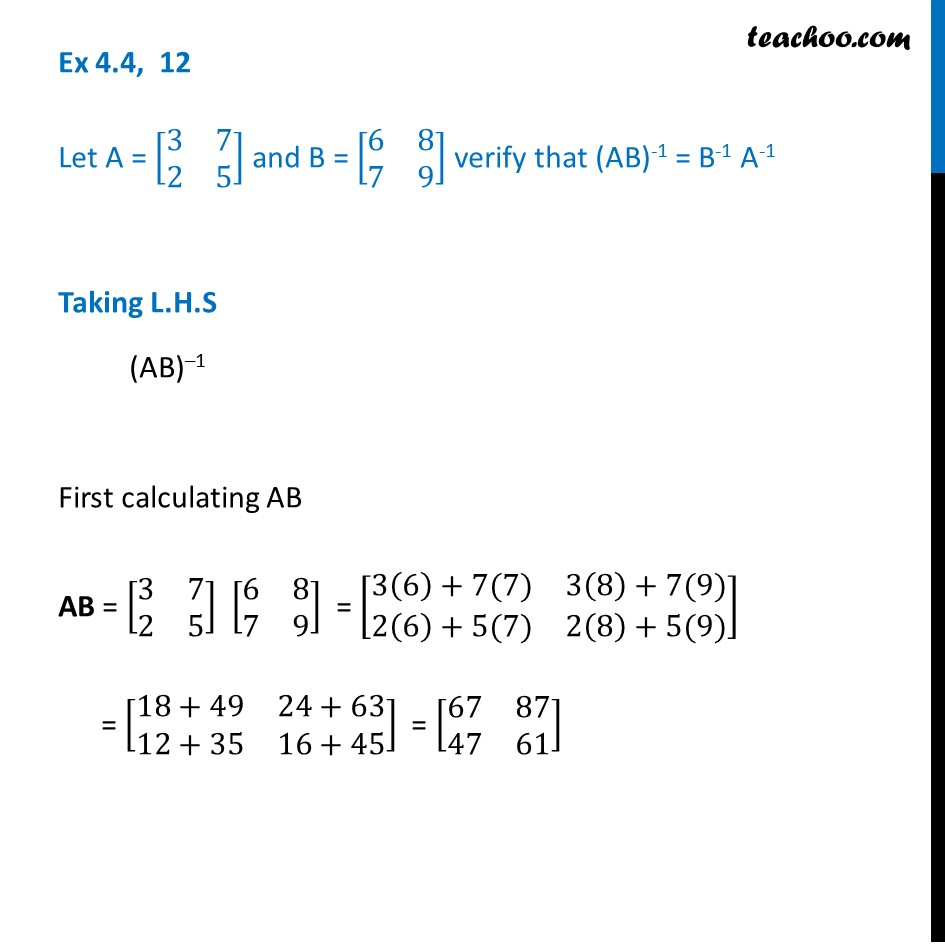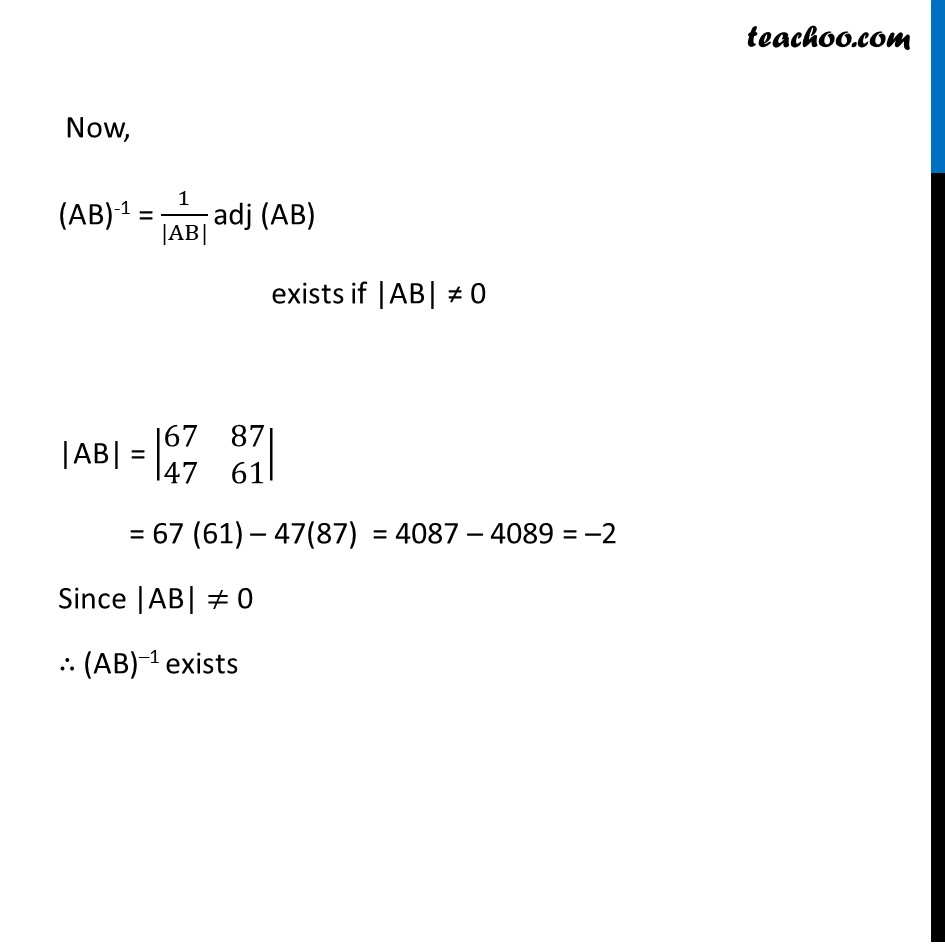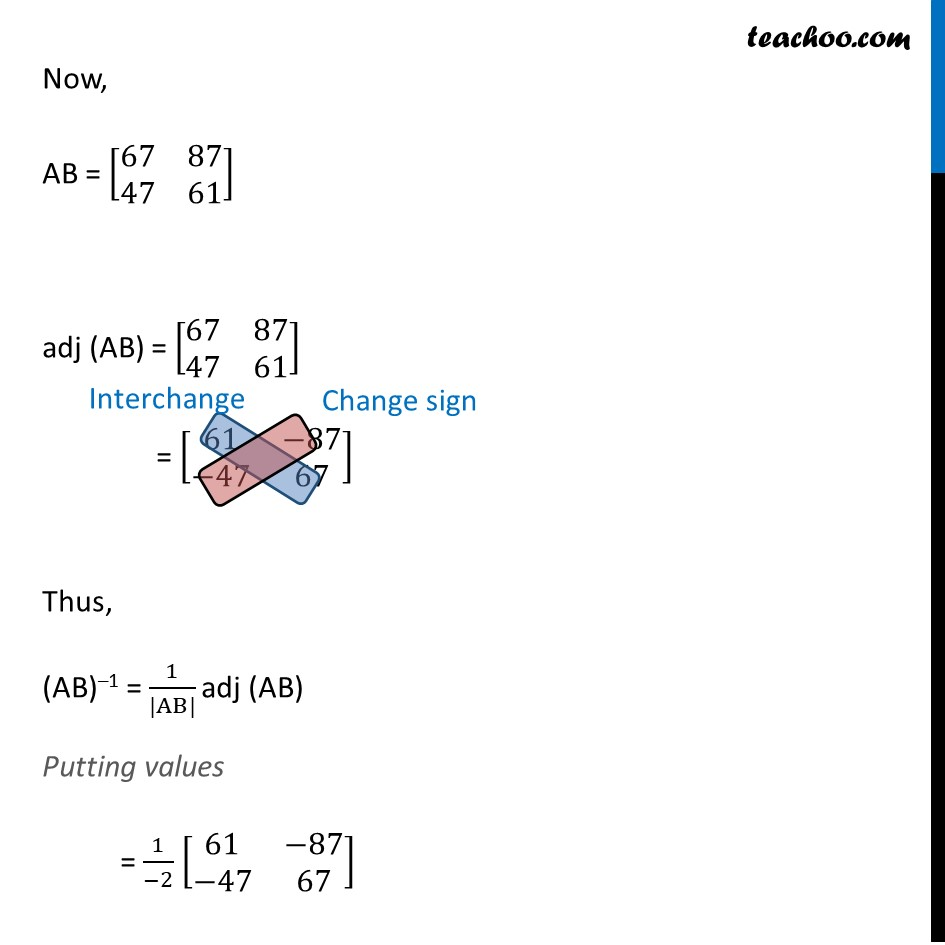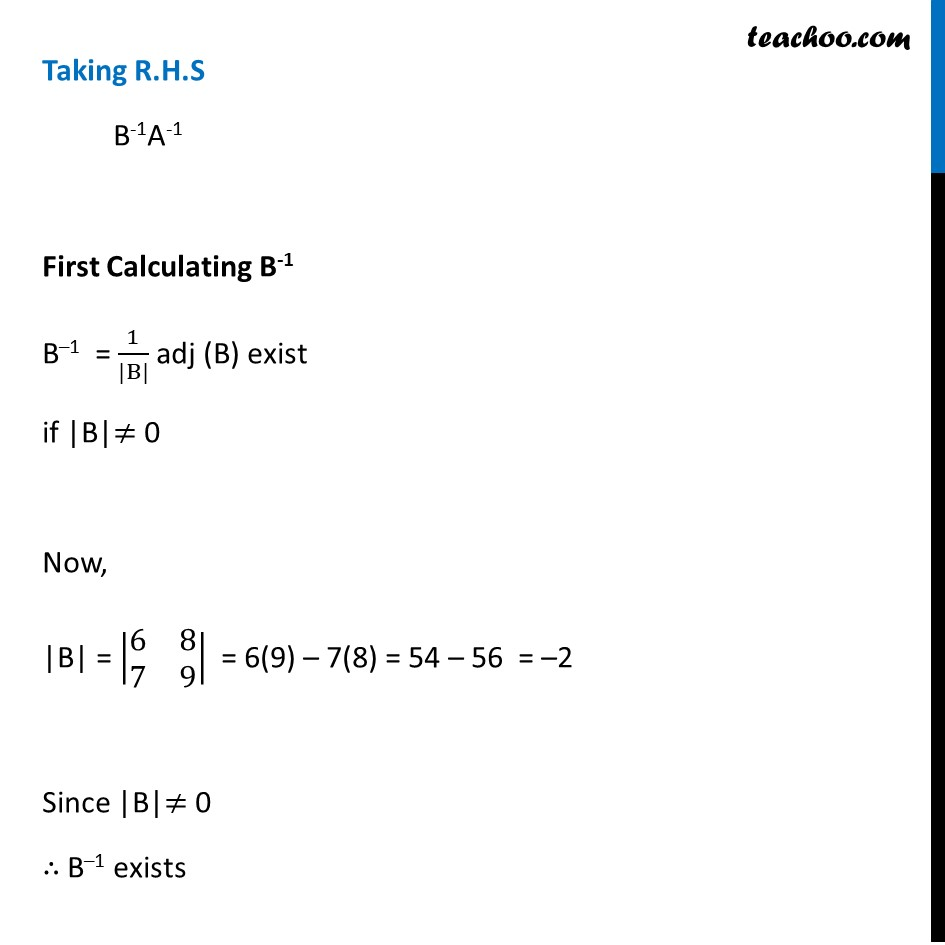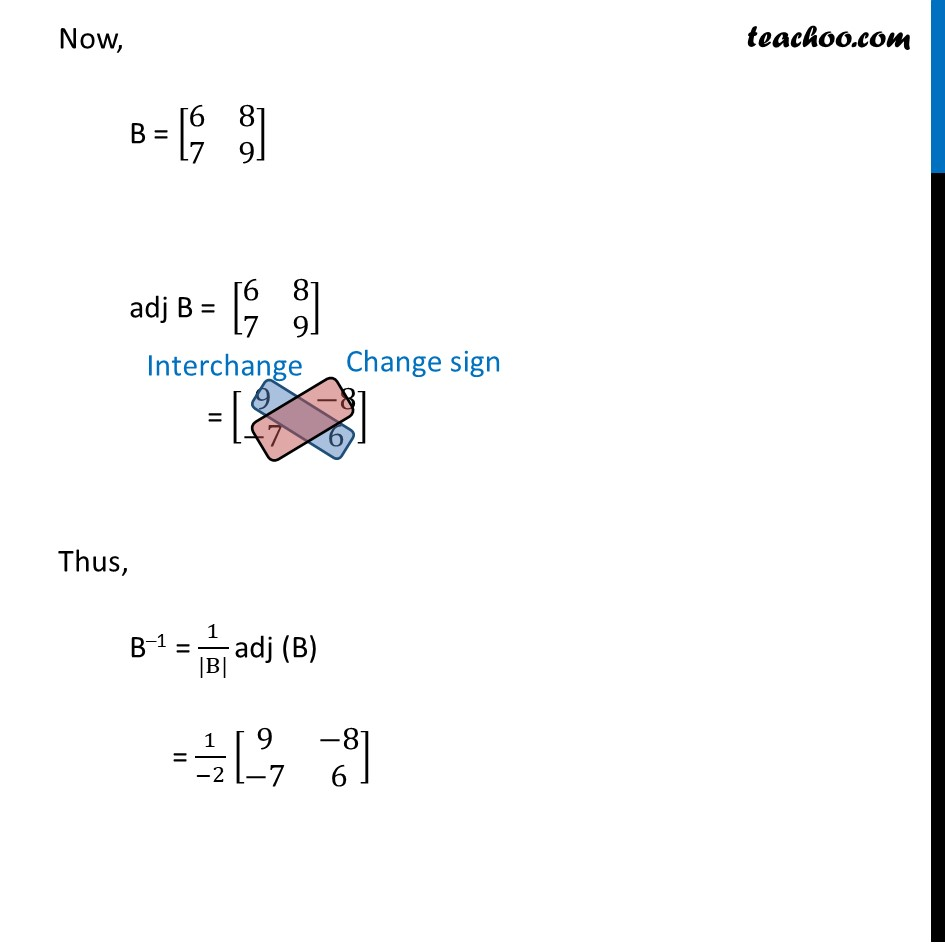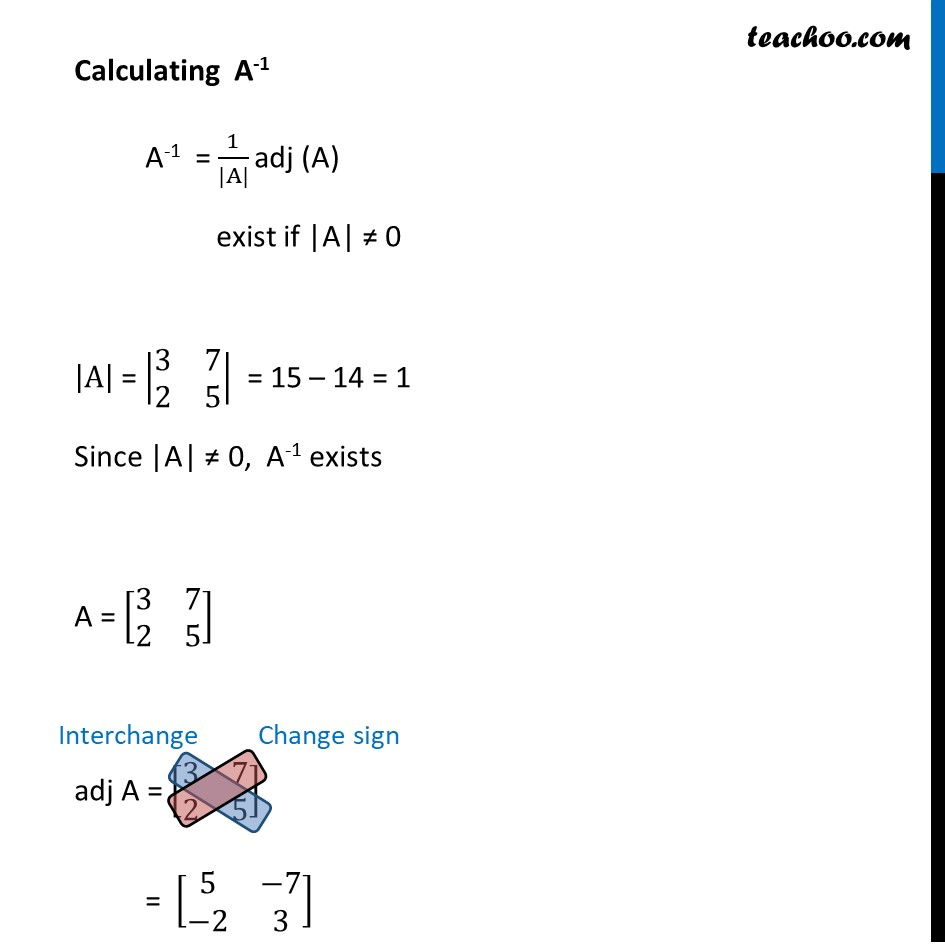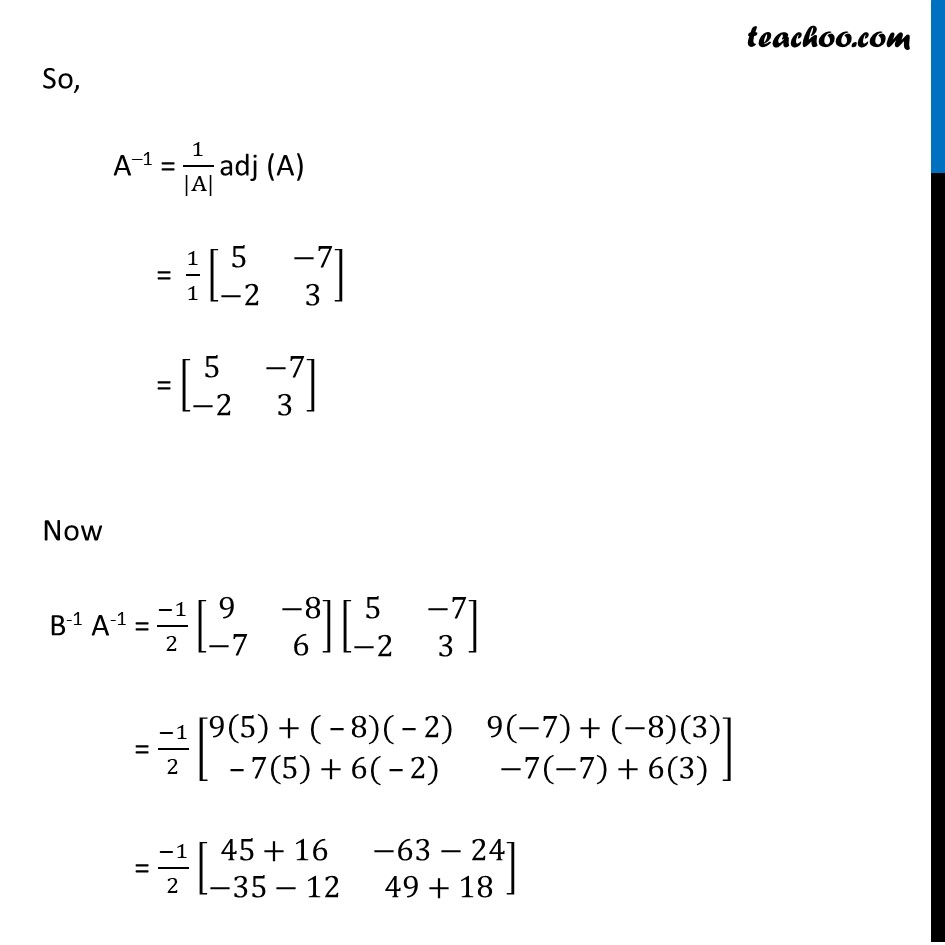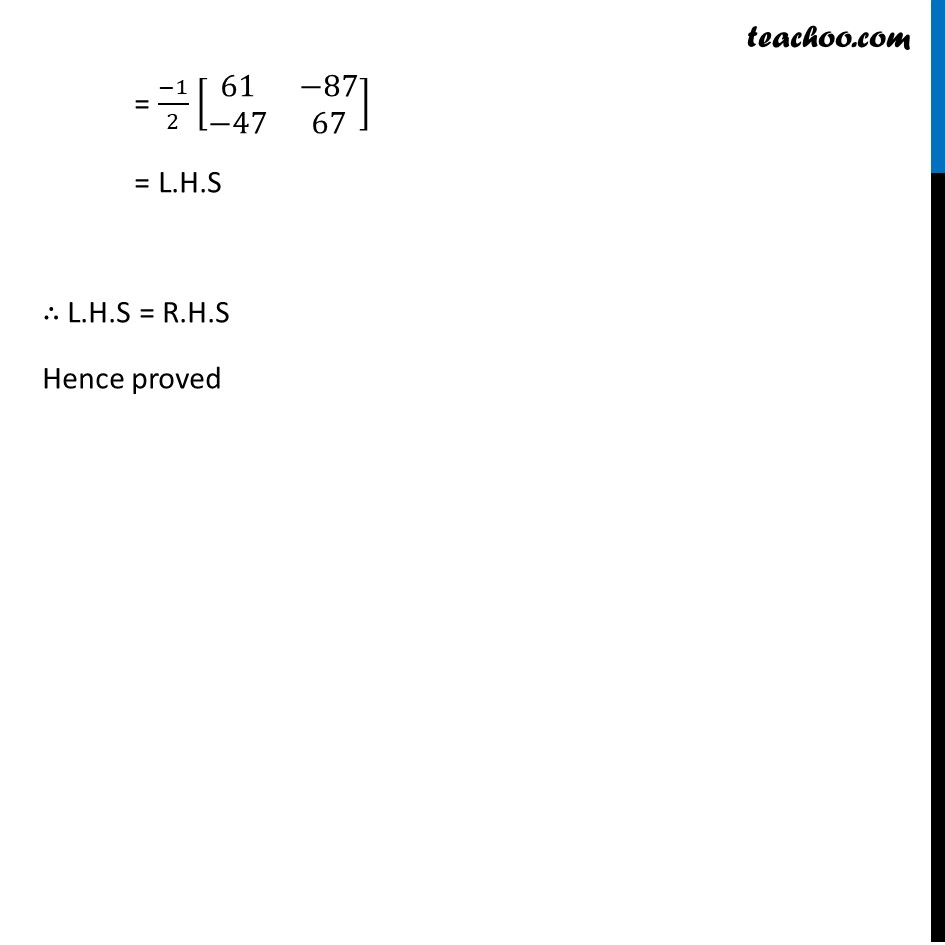Learn in your speed, with individual attention - Teachoo Maths 1-on-1 Class

### Transcript

Ex 4.4, 12 Let A = [■8(3&7@2&5)] and B = [■8(6&8@7&9)] verify that (AB)-1 = B-1 A-1 Taking L.H.S (AB)–1 First calculating AB AB = [■8(3&7@2&5)] [■8(6&8@7&9)] = [■8(3(6)+7(7)&3(8)+7(9)@2(6)+5(7)&2(8)+5(9))] = [■8(18+49&24+63@12+35&16+45)] = [■8(67&87@47&61)] Now, (AB)-1 = 1/(|AB|) adj (AB) exists if |AB| ≠ 0 |AB| = |■8(67&87@47&61)| = 67 (61) – 47(87) = 4087 – 4089 = –2 Since |AB| ≠ 0 ∴ (AB)–1 exists Now, AB = [■8(67&87@47&61)] adj (AB) = [■8(67&87@47&61)] = [■8(61&−87@−47&67)] Thus, (AB)–1 = 1/(|AB|) adj (AB) Putting values = 1/(−2) [■8(61&−87@−47&67)] Taking R.H.S B-1A-1 First Calculating B-1 B–1 = 1/(|B|) adj (B) exist if |B|≠ 0 Now, |B| = |■8(6&8@7&9)| = 6(9) – 7(8) = 54 – 56 = –2 Since |B|≠ 0 ∴ B–1 exists Now, B = [■8(6&8@7&9)] adj B = [■8(6&8@7&9)] = [■8(9&−8@−7&6)] Thus, B–1 = 1/(|B|) adj (B) = 1/(−2) [■8(9&−8@−7&6)] Calculating A-1 A-1 = 1/(|A|) adj (A) exist if |A| ≠ 0 |A| = |■8(3&7@2&5)| = 15 – 14 = 1 Since |A| ≠ 0, A-1 exists A = [■8(3&7@2&5)] adj A = [■8(3&7@2&5)] = [■8(5&−7@−2&3)] So, A–1 = 1/(|A|) adj (A) = 1/1 [■8(5&−7@−2&3)] = [■8(5&−7@−2&3)] Now B-1 A-1 = (−1)/2 [■8(9&−8@−7&6)] [■8(5&−7@−2&3)] = (−1)/2 [■8(9(5)+( –8)( –2)&9(−7)+(−8)(3)@ –7(5)+6( –2)&−7(−7)+6(3))] = (−1)/2 [■8(45+16&−63−24@−35−12&49+18)] = (−1)/2 [■8(61&−87@−47&67)] = L.H.S ∴ L.H.S = R.H.S Hence proved